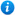# Adding Decimals (with the same number of decimal places) - Year 5

Adding Decimals (with the same number of decimal places) - Year 5

Year 5 maths programme of study - Number - fractions (including decimals)

• solve problems involving number up to three decimal places

Year 5 maths programme of study - Measurement

• use all four operations to solve problems involving measure using decimal notation

'Adding Decimals (with the same number of decimal places) - Year 5' is a KS2 maths teaching resource ideal to use in a lesson covering the year 5 curriculum objectives listed above. Content includes:

• Adding decimals with the same decimal places using place value charts activity and worksheet (no exchanges)
• Adding decimals with the same decimal places using place value charts activity and worksheet (with exchanges)
• Adding decimals with the same decimal places column addition activity and worksheet (no exchanges)
• Adding decimals with the same decimal places column addition activity and worksheet (with 1 exchange)
• Adding decimals with the same decimal places column addition activity and worksheet (with more than 1 exchange)
• Differentiated money addition problems x 3 and accompanying worksheets

'Adding Decimals (with the same number of decimal places) - Year 5' is fully editable so teachers are able to adapt the resource to suit the needs of each class they teach.

'Adding Decimals (with the same number of decimal places) - Year 5' can be previewed in full by clicking on the PowerPoint images.

Our Price : £2.99 / 3 Credits